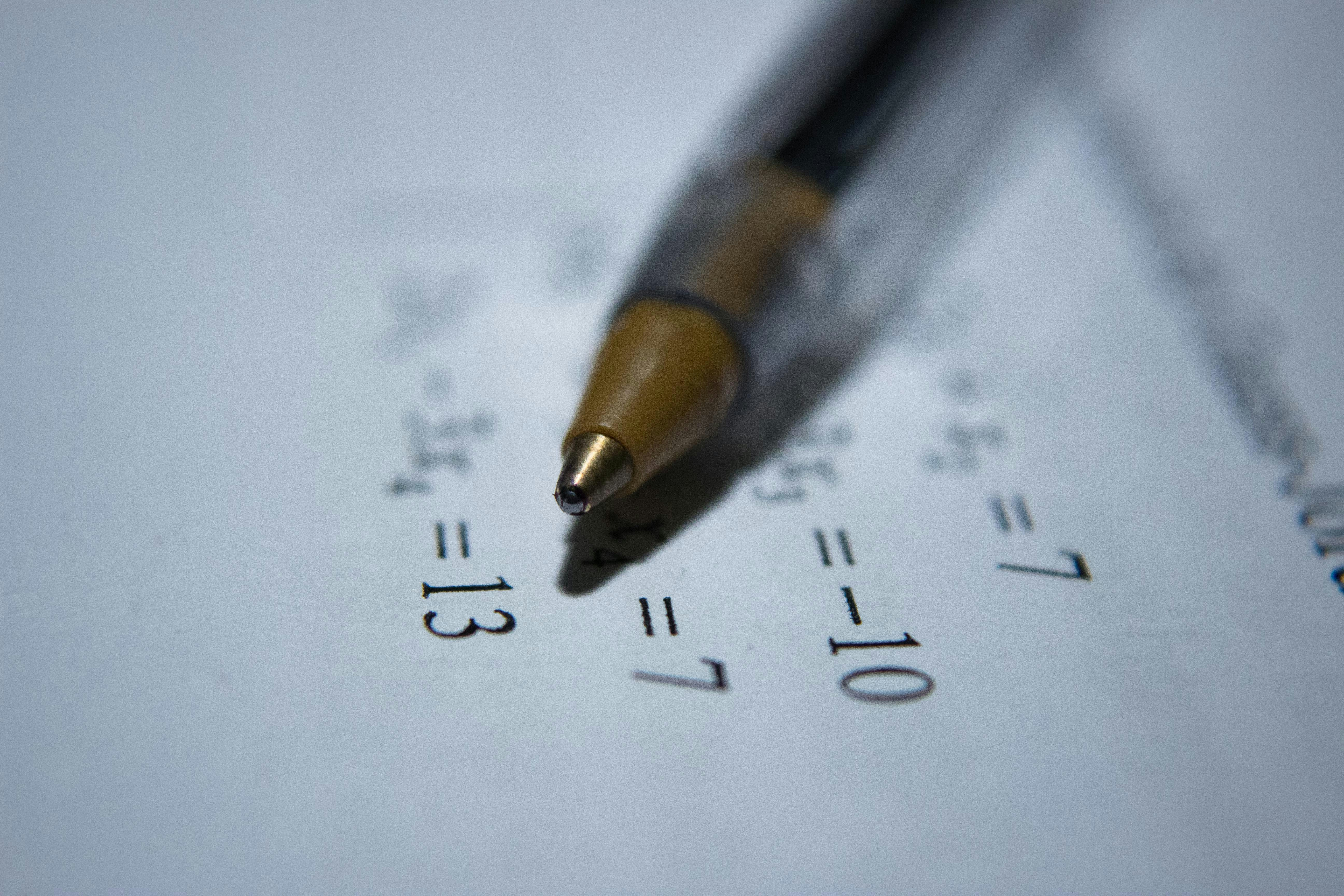Math is one of the most hated subjects in a student’s life. Understanding its concepts and solving mathematical equations may feel like a daunting task for many pupils. However, understanding simple math facts can offer advantages in schools and may develop students’ interest in this subject. Students can be taught to solve simple addition and subtraction problems mentally through mental math. It improves their math fluency and they can move from basic to advanced levels easily. Parents and teachers can help students develop their mental math skills through simple math games.

## What is the mental math method?

The mental math method refers to solving a mathematical problem mentally without writing it down on a piece of paper. Through this technique, students can learn to carry out addition, subtraction, and other mental calculations. It helps them solve a problem without whipping out a calculator or using a pen and paper. This technique is best suited to develop problem-solving skills in students. Moreover, it also makes students see the relationship between numbers and patterns they make. When students practice mental math continuously, they learn a few crucial skills listed below.

• Remembering facts

When practicing mental calculations, students learn the facts crucial for solving mathematical equations. These facts can help them move from basic to advanced levels in a few weeks or months. However, regular practice is required to get the most out of mental math sessions.

• Estimating calculations

Mental math allows students to learn the ability to estimate the answer. Students can learn to round the number up and down before solving the equation mentally.

• Mathematical speed

Speed and accuracy are crucial when solving mathematical equations. These qualities help students solve more questions in less time.

Students can learn mental math abilities by practicing more questions. However, they are better able to use this concept in practice with the help of games. Let us look at a few simple games and how teachers can use these games to make students learn the art of mental calculations.

## Games to improve mental math abilities

Here are a few simple but effective games that can improve students' mental math abilities.

### Multiplication War

It is a card game that improves the multiplication facts of students. Teachers can shuffle a deck of cards and give each player an equal number. They can assign the picture cards a value of 10 and aces a value of 11. Each player draws two cards, reads the number sentence, and gives an answer. For example, if a player draws cards having numbers 6 and 4, they get 24 points. Likewise, another player drawing cards having a 7 and a 6 would have received 42. Students getting larger products win the cards and keep them at the bottom of their pile. Players can continue the game until their opponent runs out of cards.

### Mental Mystery

The mental mystery is the best game to make students learn simple subtraction. Teachers can ask students to collect as many tiny objects as they can. Students can collect marbles and candies for this game. Once they have collected different objects, they need to close their eyes in the next step. Teachers can hide some of the objects and ask students to count the rest. This activity can be continued until students can solve these simple subtraction problems easily.

### 101 and Out

Collect a sheet of paper, a pencil, and dice to play this game. In this game, students need to score as close to 101 without going over. Students can roll the dice and write the number on the sheet. In the final step, they can add all the numbers to see their final score.

### Math Jeopardy

Teachers can say a number aloud and ask students to write that number on a piece of paper. In the next few minutes, students need to find as many ways as they can to make the number using addition, subtraction, multiplication, and division.

These games make mental math fun for students. It improves their calculation and makes them focus on their mathematical abilities. Learning some basic mental math abilities can help students improve their self-confidence.

## How do you practice mental maths?

Following the tips mentioned below can help students find the answers to mathematical problems in no time.

• Breaking addition and subtraction problems into parts
• Changing the equation to bring it close to round numbers
• Reordering the numbers to make the sums convenient to solve
• Multiplying from left to right
• Breaking problems into parts to turn a complex problem into two easier ones
• Simplifying problems with numbers ending in zero
• Memorizing the 11s tricks
• Turning percentages into easy-to-solve problems
• Remembering square charts
• Practicing mental math daily

Mental math can be mastered by following the tips mentioned above. Mastering this ability improves problem-solving skills and makes students retain the information they find crucial. Games are one of the best ways to teach this calculation technique to students.

Teachmint is one of the leading education infrastructure providers in the country. With our advanced learning management system, you can improve the teaching-learning experience. Our offerings like education erp, admission management system, fee management system, and others conveniently digitize educational institutions.

Suggested Read: The Social Responsibility of Being a Teacher

Name must have atleast 3 characters
School name must have atleast 3 characters
Phone number must have atleast 7 digits and atmost 15 digits